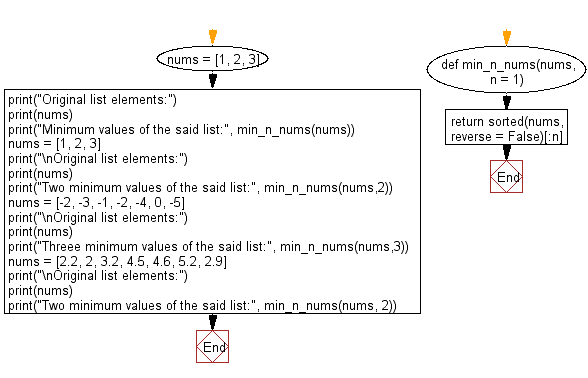﻿ Python: Find the n minimum elements from the provided list - w3resource# Python: Find the n minimum elements from the provided list

## Python List: Exercise - 253 with Solution

Write a Python program to get the n minimum elements from a given list of numbers.

• Use sorted() to sort the list.
• Use slice notation to get the specified number of elements.
• Omit the second argument, n, to get a one-element list.
• If n is greater than or equal to the provided list's length, then return the original list (sorted in ascending order).

Sample Solution:

Python Code:

``````def min_n_nums(nums, n = 1):
return sorted(nums, reverse = False)[:n]

nums = [1, 2, 3]
print("Original list elements:")
print(nums)
print("Minimum values of the said list:", min_n_nums(nums))
nums = [1, 2, 3]
print("\nOriginal list elements:")
print(nums)
print("Two minimum values of the said list:", min_n_nums(nums,2))
nums = [-2, -3, -1, -2, -4, 0, -5]
print("\nOriginal list elements:")
print(nums)
print("Threee minimum values of the said list:", min_n_nums(nums,3))
nums = [2.2, 2, 3.2, 4.5, 4.6, 5.2, 2.9]
print("\nOriginal list elements:")
print(nums)
print("Two minimum values of the said list:", min_n_nums(nums, 2))
```
```

Sample Output:

```Original list elements:
[1, 2, 3]
Minimum values of the said list: 

Original list elements:
[1, 2, 3]
Two minimum values of the said list: [1, 2]

Original list elements:
[-2, -3, -1, -2, -4, 0, -5]
Threee minimum values of the said list: [-5, -4, -3]

Original list elements:
[2.2, 2, 3.2, 4.5, 4.6, 5.2, 2.9]
Two minimum values of the said list: [2, 2.2]
```

Flowchart:## Visualize Python code execution:

The following tool visualize what the computer is doing step-by-step as it executes the said program:

Python Code Editor:

Have another way to solve this solution? Contribute your code (and comments) through Disqus.

What is the difficulty level of this exercise?

Test your Python skills with w3resource's quiz

﻿

## Python: Tips of the Day

```print(2_000_000)
```2000000